## 用Numpy实现简单的神经网络

article/2023/9/24 22:56:55

### 目录

• 数据预处理
• 模型设计
• 训练过程
• 小批量随机梯度下降

M S E = 1 N ∑ i = 1 N ( Y i ^ − Y i ) 2 MSE = \frac{1}{N} \sum_{i=1}^N(\hat{Yi} - {Y_i})^{2}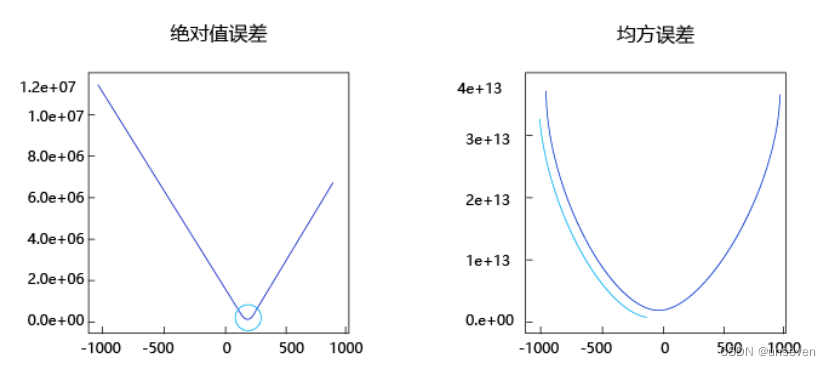• 曲线的最低点是可导的。
• 越接近最低点，曲线的坡度逐渐放缓，有助于通过当前的梯度来判断接近最低点的程度（是否逐渐减少步长，以免错过最低点）。

## 数据预处理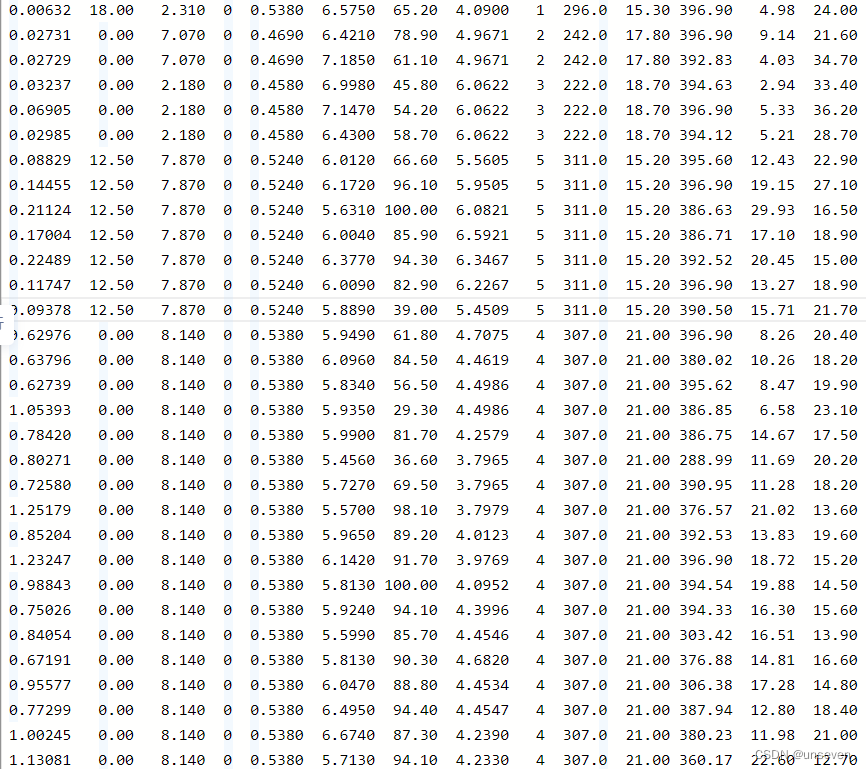# 导入需要用到的package
import numpy as np
import json
# 读入训练数据
datafile = './work/housing.data'
data = np.fromfile(datafile, sep=' ')
data.shape
--------------------------------
(7084,)


feature_names = [ 'CRIM', 'ZN', 'INDUS', 'CHAS', 'NOX', 'RM', 'AGE','DIS', 'RAD', 'TAX', 'PTRATIO', 'B', 'LSTAT', 'MEDV' ]
feature_num = len(feature_names)
data = data.reshape([data.shape // feature_num, feature_num])
data.shape
--------------------------------------
(506, 14)


ratio = 0.8
offset = int(data.shape * ratio)
training_data = data[:offset]
training_data.shape
-----------------------------------
(404, 14)


# 计算train数据集的最大值，最小值
maximums, minimums = training_data.max(axis=0), \training_data.min(axis=0),
# 对数据进行归一化处理
for i in range(feature_num):data[:, i] = (data[:, i] - minimums[i]) / (maximums[i] - minimums[i])


def load_data():# 从文件导入数据datafile = './work/housing.data'data = np.fromfile(datafile, sep=' ')# 每条数据包括14项，其中前面13项是影响因素，第14项是相应的房屋价格中位数feature_names = [ 'CRIM', 'ZN', 'INDUS', 'CHAS', 'NOX', 'RM', 'AGE', \'DIS', 'RAD', 'TAX', 'PTRATIO', 'B', 'LSTAT', 'MEDV' ]feature_num = len(feature_names)# 将原始数据进行Reshape，变成[N, 14]这样的形状data = data.reshape([data.shape // feature_num, feature_num])# 将原数据集拆分成训练集和测试集# 这里使用80%的数据做训练，20%的数据做测试# 测试集和训练集必须是没有交集的ratio = 0.8offset = int(data.shape * ratio)training_data = data[:offset]# 计算训练集的最大值，最小值maximums, minimums = training_data.max(axis=0), \training_data.min(axis=0)# 对数据进行归一化处理for i in range(feature_num):data[:, i] = (data[:, i] - minimums[i]) / (maximums[i] - minimums[i])# 训练集和测试集的划分比例training_data = data[:offset]test_data = data[offset:]return training_data, test_data


# 获取数据
x = training_data[:, :-1]
y = training_data[:, -1:]


## 模型设计

class Network(object):def __init__(self, num_of_weights):# 随机产生w的初始值# 为了保持程序每次运行结果的一致性，# 此处设置固定的随机数种子np.random.seed(0)self.w = np.random.randn(num_of_weights, 1)self.b = 0.def forward(self, x):z = np.dot(x, self.w) + self.breturn z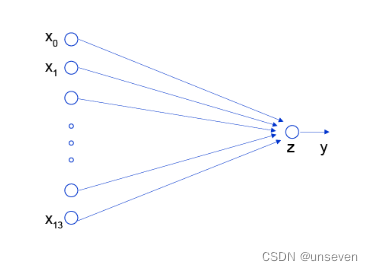L o s s = ( y − z ) 2 Loss = (y - z)^2

L o s s = 1 N ∑ i = 1 N ( y i − z i ) 2 Loss= \frac{1}{N}\sum_{i=1}^N{(y_i - z_i)^2}

class Network(object):def __init__(self, num_of_weights):# 随机产生w的初始值# 为了保持程序每次运行结果的一致性，此处设置固定的随机数种子np.random.seed(0)self.w = np.random.randn(num_of_weights, 1)self.b = 0.def forward(self, x):z = np.dot(x, self.w) + self.breturn zdef loss(self, z, y):error = z - ycost = error * errorcost = np.mean(cost)return cost


net = Network(13)
# 可以一次性计算多个样本的预测值和损失函数
x1 = x[0:3]
y1 = y[0:3]
z = net.forward(x1)
print('predict: ', z)
loss = net.loss(z, y1)
print('loss:', loss)
------------------------------------------------------
predict:  [[2.39362982][2.46752393][2.02483479]]
loss: 3.384496992612791


## 训练过程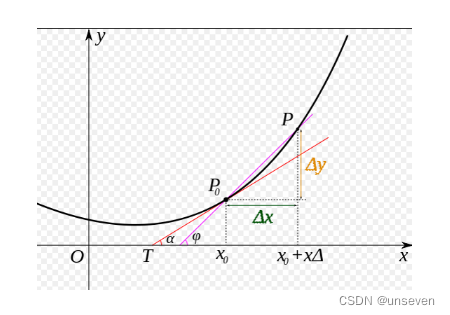∂ L ∂ w = 0 \frac{\partial{L}}{\partial{w}}=0
∂ L ∂ b = 0 \frac{\partial{L}}{\partial{b}}=0

L = 1 2 N ∑ i = 1 N ( y i − z i ) 2 L= \frac{1}{2N}\sum_{i=1}^N{(y_i - z_i)^2}

z i = ∑ j = 0 12 x i j ⋅ w j + b z_i = \sum_{j=0}^{12}{x_i^{j}\cdot w_j} + b

g r a d i e n t = ( ∂ L ∂ w 0 , ∂ L ∂ w 1 , . . . , ∂ L ∂ w 12 , ∂ L ∂ b ) gradient= (\frac{\partial{L}}{\partial{w_0}},\frac{\partial{L}}{\partial{w_1}}, ... ,\frac{\partial{L}}{\partial{w_{12}}} ,\frac{\partial{L}}{\partial{b}})

∂ L ∂ w j = 1 N ∑ i = 1 N ( z i − y i ) ∂ z i ∂ w j = 1 N ∑ i = 1 N ( z i − y i ) x i j \frac{\partial{L}}{\partial{w_j}} = \frac{1}{N}\sum_{i=1}^N{(z_i - y_i)\frac{\partial{z_i}}{\partial{w_j}}} = \frac{1}{N}\sum_{i=1}^N{(z_i - y_i)x_i^{j}}

∂ L ∂ b = 1 N ∑ i = 1 N ( z i − y i ) ∂ z i ∂ b = 1 N ∑ i = 1 N ( z i − y i ) \frac{\partial{L}}{\partial{b}} = \frac{1}{N}\sum_{i=1}^N{(z_i - y_i)\frac{\partial{z_i}}{\partial{b}}} = \frac{1}{N}\sum_{i=1}^N{(z_i - y_i)}

L = 1 2 ( y i − z i ) 2 L= \frac{1}{2}{(y_i - z_i)^2}

z 1 = x 1 0 ⋅ w 0 + x 1 1 ⋅ w 1 + . . . + x 1 12 ⋅ w 12 + b z_1 = {x_1^{0}\cdot w_0} + {x_1^{1}\cdot w_1} + ... + {x_1^{12}\cdot w_{12}} + b

L = 1 2 ( x 1 0 ⋅ w 0 + x 1 1 ⋅ w 1 + . . . + x 1 12 ⋅ w 12 + b − y 1 ) 2 L= \frac{1}{2}{({x_1^{0}\cdot w_0} + {x_1^{1}\cdot w_1} + ... + {x_1^{12}\cdot w_{12}} + b - y_1)^2}

∂ L ∂ w 0 = ( x 1 0 ⋅ w 0 + x 1 1 ⋅ w 1 + . . . + x 1 12 ⋅ w 1 2 + b − y 1 ) ⋅ x 1 0 = ( z 1 − y 1 ) ⋅ x 1 0 \frac{\partial{L}}{\partial{w_0}} = ({x_1^{0}\cdot w_0} + {x_1^{1}\cdot w_1} + ... + {x_1^{12}\cdot w_12} + b - y_1)\cdot x_1^{0}=({z_1} - {y_1})\cdot x_1^{0}

∂ L ∂ b = ( x 1 0 ⋅ w 0 + x 1 1 ⋅ w 1 + . . . + x 1 12 ⋅ w 12 + b − y 1 ) ⋅ 1 = ( z 1 − y 1 ) \frac{\partial{L}}{\partial{b}} = ({x_1^{0}\cdot w_0} + {x_1^{1}\cdot w_1} + ... + {x_1^{12}\cdot w_{12}} + b - y_1)\cdot 1 = ({z_1} - {y_1})

# 注意这里是一次取出3个样本的数据，不是取出第3个样本
x3samples = x[0:3]
y3samples = y[0:3]
z3samples = net.forward(x3samples)
gradient_w = (z3samples - y3samples) * x3samples


∂ L ∂ w j = 1 N ∑ i = 1 N ( z i − y i ) ∂ z i ∂ w j = 1 N ∑ i = 1 N ( z i − y i ) x i j \frac{\partial{L}}{\partial{w_j}} = \frac{1}{N}\sum_{i=1}^N{(z_i - y_i)\frac{\partial{z_i}}{\partial{w_j}}} = \frac{1}{N}\sum_{i=1}^N{(z_i - y_i)x_i^{j}}

z = net.forward(x)
gradient_w = (z - y) * x
# axis = 0 表示把每一行做相加然后再除以总的行数


gradient_b = (z - y)
# 此处b是一个数值，所以可以直接用np.mean得到一个标量


class Network(object):def __init__(self, num_of_weights):# 随机产生w的初始值# 为了保持程序每次运行结果的一致性，此处设置固定的随机数种子np.random.seed(0)self.w = np.random.randn(num_of_weights, 1)self.b = 0.def forward(self, x):z = np.dot(x, self.w) + self.breturn zdef loss(self, z, y):error = z - ynum_samples = error.shapecost = error * errorcost = np.sum(cost) / num_samplesreturn costdef gradient(self, x, y):z = self.forward(x)gradient_w = (z-y)*xgradient_w = np.mean(gradient_w, axis=0)gradient_w = gradient_w[:, np.newaxis]gradient_b = (z - y)gradient_b = np.mean(gradient_b)return gradient_w, gradient_b


• 相减：参数需要向梯度的反方向移动。
• eta：控制每次参数值沿着梯度反方向变动的大小，即每次移动的步长，又称为学习率。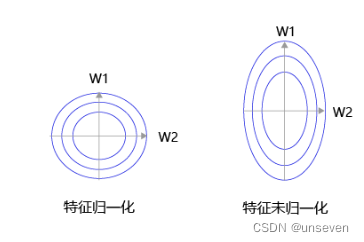class Network(object):def __init__(self, num_of_weights):# 随机产生w的初始值# 为了保持程序每次运行结果的一致性，此处设置固定的随机数种子np.random.seed(0)self.w = np.random.randn(num_of_weights, 1)self.b = 0.def forward(self, x):z = np.dot(x, self.w) + self.breturn zdef loss(self, z, y):error = z - ynum_samples = error.shapecost = error * errorcost = np.sum(cost) / num_samplesreturn costdef gradient(self, x, y):z = self.forward(x)gradient_w = (z-y)*xgradient_w = np.mean(gradient_w, axis=0)gradient_w = gradient_w[:, np.newaxis]gradient_b = (z - y)gradient_b = np.mean(gradient_b)        return gradient_w, gradient_bdef update(self, gradient_w, gradient_b, eta = 0.01):self.w = self.w - eta * gradient_wself.b = self.b - eta * gradient_bdef train(self, x, y, iterations=100, eta=0.01):losses = []for i in range(iterations):z = self.forward(x)L = self.loss(z, y)gradient_w, gradient_b = self.gradient(x, y)self.update(gradient_w, gradient_b, eta)losses.append(L)if (i+1) % 10 == 0:print('iter {}, loss {}'.format(i, L))return losses# 获取数据
x = train_data[:, :-1]
y = train_data[:, -1:]
# 创建网络
net = Network(13)
num_iterations=1000
# 启动训练
losses = net.train(x,y, iterations=num_iterations, eta=0.01)# 画出损失函数的变化趋势
plot_x = np.arange(num_iterations)
plot_y = np.array(losses)
plt.plot(plot_x, plot_y)
plt.show()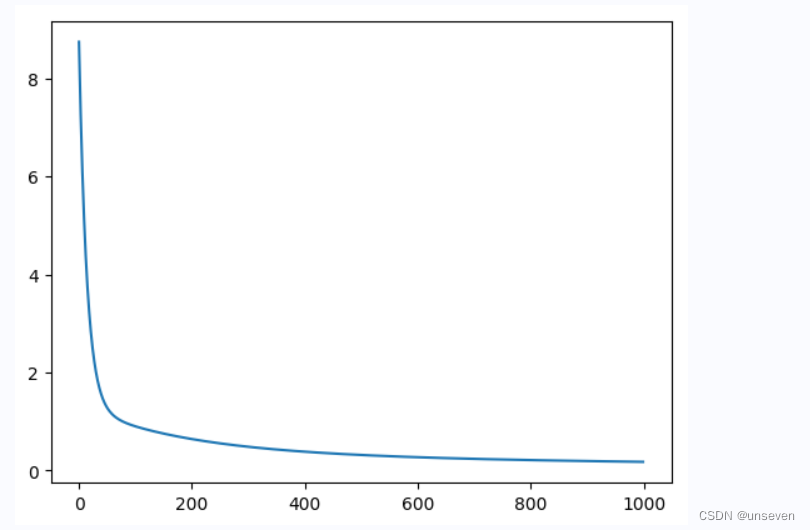## 小批量随机梯度下降

• minibatch：每次迭代时抽取出来的一批数据被称为一个minibatch。
• batch size：每个minibatch所包含的样本数目称为batch size。
• Epoch：当程序迭代的时候，按minibatch逐渐抽取出样本，当把整个数据集都遍历到了的时候，则完成了一轮训练，也叫一个Epoch（轮次）。启动训练时，可以将训练的轮数num_epochs和batch_size作为参数传入。

a = np.array([1,2,3,4,5,6,7,8,9,10,11,12])
print('before shuffle', a)
np.random.shuffle(a)
print('after shuffle', a)
-------------------------------------
before shuffle [ 1  2  3  4  5  6  7  8  9 10 11 12]
after shuffle [ 7  2 11  3  8  6 12  1  4  5 10  9]


import numpy as npclass Network(object):def __init__(self, num_of_weights):# 随机产生w的初始值# 为了保持程序每次运行结果的一致性，此处设置固定的随机数种子#np.random.seed(0)self.w = np.random.randn(num_of_weights, 1)self.b = 0.def forward(self, x):z = np.dot(x, self.w) + self.breturn zdef loss(self, z, y):error = z - ynum_samples = error.shapecost = error * errorcost = np.sum(cost) / num_samplesreturn costdef gradient(self, x, y):z = self.forward(x)N = x.shapegradient_w = 1. / N * np.sum((z-y) * x, axis=0)gradient_w = gradient_w[:, np.newaxis]gradient_b = 1. / N * np.sum(z-y)return gradient_w, gradient_bdef update(self, gradient_w, gradient_b, eta = 0.01):self.w = self.w - eta * gradient_wself.b = self.b - eta * gradient_bdef train(self, training_data, num_epochs, batch_size=10, eta=0.01):n = len(training_data)losses = []for epoch_id in range(num_epochs):# 在每轮迭代开始之前，将训练数据的顺序随机打乱# 然后再按每次取batch_size条数据的方式取出np.random.shuffle(training_data)# 将训练数据进行拆分，每个mini_batch包含batch_size条的数据mini_batches = [training_data[k:k+batch_size] for k in range(0, n, batch_size)]for iter_id, mini_batch in enumerate(mini_batches):#print(self.w.shape)#print(self.b)x = mini_batch[:, :-1]y = mini_batch[:, -1:]a = self.forward(x)loss = self.loss(a, y)gradient_w, gradient_b = self.gradient(x, y)self.update(gradient_w, gradient_b, eta)losses.append(loss)print('Epoch {:3d} / iter {:3d}, loss = {:.4f}'.format(epoch_id, iter_id, loss))return losses# 获取数据
net = Network(13)
# 启动训练
losses = net.train(train_data, num_epochs=50, batch_size=100, eta=0.1)# 画出损失函数的变化趋势
plot_x = np.arange(len(losses))
plot_y = np.array(losses)
plt.plot(plot_x, plot_y)
plt.show()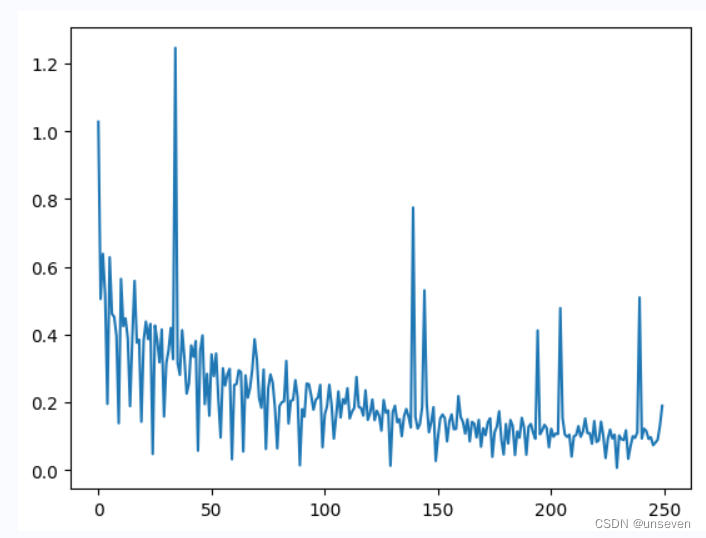### Docker基础入门

docker基础教程&#xff1a; 第一章&#xff1a;docker与虚拟机的区别 docker与虚拟机的主要区别在于docker是一个在你本机操作系统中独立运行的一个进程&#xff0c;而虚拟机则是一个完整的隔离的操作系统&#xff0c;它运行在你本机操作系统之上&#xff0c;需要更多时间和…

### 中级软件设计师考试经验总结

2023年5月&#xff0c;中级软考终于结束了&#xff0c;对了一下答案&#xff0c;确定的分上午大概55分左右&#xff0c;下午大概55分左右&#xff0c;应该通过是没有问题的。备考时间大概不到1个月吧&#xff0c;中途公司的事也非常忙&#xff0c;只能周末抽出大段时间复习&…

### chatgpt赋能python：Python反转字符串——简单易学的编程技巧

Python反转字符串——简单易学的编程技巧 你是否曾经遇到过需要反转字符串的情况&#xff1f;例如&#xff0c;你想把“Hello World”转换成“dlroW olleH”&#xff1f;如果是这样&#xff0c;Python可以帮你完成这个任务。在本文中&#xff0c;我们将看到如何使用Python的简…

### 【数据仓库架构】什么是 Azure Synapse，它与 Azure Data Bricks 有何不同？

Azure Synapse Analytics 是一项针对大型公司的无限信息分析服务&#xff0c;它被呈现为 Azure SQL 数据仓库 (SQL DW) 的演变&#xff0c;将业务数据存储和宏或大数据分析结合在一起。 在处理、管理和提供数据以满足即时商业智能和数据预测需求时&#xff0c;Synapse 为所有工…